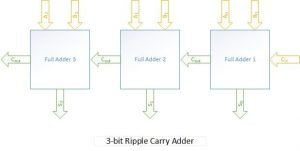Basic gates such as NOT, OR, AND, NAND and NOR are used to carry out basic arithmetic operations like addition, subtraction, multiplication and division in processors. Addition is the most basic arithmetic operation, as subtraction (a series of negative addition), multiplication (a series of positive addition) and division (a series of negative addition) can be carried out as an extended operation of simple addition.

The simple adder module without carry in is called half adder and the adder with carry in is called full adder. Sample verilog code for a half adder and full adder can be found below.

[cc lang=”verilog” escaped=true]

output S,Cout,
input A,B
);

assign S = A^B;
assign Cout = A*B;

endmodule

output S,Cout,
input A,B,Cin
);

assign S = (A^B) ^ Cin;
assign Cout = (A*B) | (A^B)*Cin;

endmodule

[/cc]

[cc lang=”verilog” ]

output reg [0:2] S,
output reg Cout,
input [0:2] A,B
);

reg [0:1] c;